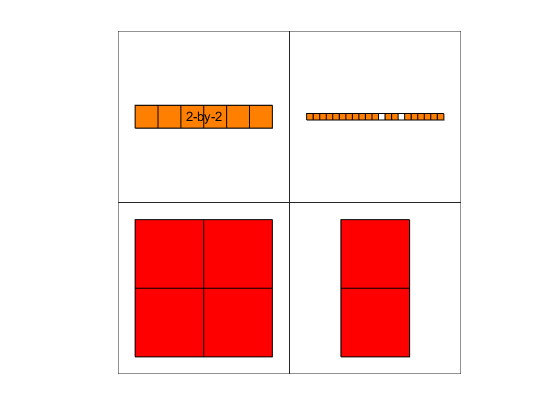# cellplot

Graphically display structure of cell array

## Syntax

```cellplot(c) cellplot(c, 'legend') handles = cellplot(c) ```

## Description

`cellplot(c)` displays a figure window that graphically represents the contents of `c`. Filled rectangles represent elements of vectors and arrays, while scalars and short character vectors are displayed as text.

`cellplot(c, 'legend')` places a colorbar next to the plot labelled to identify the data types in `c`.

`handles = cellplot(c)` displays a figure window and returns a vector of surface handles.

## Limitations

The `cellplot` function can display only two-dimensional cell arrays.

## Examples

Consider a 2-by-2 cell array containing a matrix, a vector, and two character vectors:

```c{1,1} = '2-by-2'; c{1,2} = 'eigenvalues of eye(2)'; c{2,1} = eye(2); c{2,2} = eig(eye(2));```

The command `cellplot(c)` produces## Version History

Introduced before R2006a